Next: Shaping a ghost to Up: SHAPING FILTER Previous: SHAPING FILTER

## Source waveform and multiple reflections

Figure 1 shows some reflection seismic data recorded at nearly vertical incidence from an arctic ice sheet.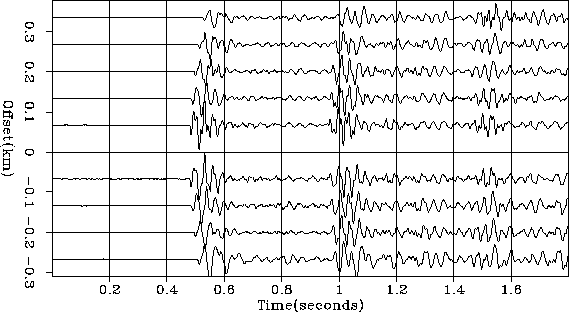wz24
Figure 1
Some of the inner offset seismograms from Arctic dataset 24 (Yilmaz and Cumro).

Apparently the initial waveform is somewhat complex, but the water-bottom reflection does not complicate it further. You can confirm this by noticing the water-bottom multiple reflection, i.e., the wave that bounces first from the water bottom, then from the water surface, and then a second time from the water bottom. This multiple reflection is similar to but has polarity opposite to the shape of the primary water-bottom reflection. (The opposite polarity results from the reflection at the ocean surface, where the acoustic pressure, the sum of the downgoing wave plus the upgoing wave, vanishes.)

Other data in water of similar depth shows a different reflection behavior. The bottom gives back not a single reflection, but a train of reflections. Let this train of reflections from the ocean floor be denoted by F(Z). Instead of looking like -F(Z), the first multiple reflection can look like -F(Z)2. The ray sketch in Figure 2 shows a simple multiple reflection.

 peg Figure 2 Water bottom soft-mud multiple (left) and similar travel times to mudstone (center and right).There is only one water-bottom path, but there are two paths to a slightly deeper layer. I will call the first arrival the soft-mud arrival and the second one the mudstone arrival. If these two arrivals happen to have the same strength, an expression for F(Z) is 1+Z. The expression for the first multiple is -F(Z)2=-(1+Z)2=-1+2Z-Z2 where the 2Z represents the two paths in Figure 2. The waveform of the second water bottom multiple is (1-Z)3 in which the mudstone would be three times as strong as the soft mud. In the nth wave train the mudstone is n times as strong as the soft mud. Figure 3 is a textbook quality example of this simple concept.wz30
Figure 3
Two displays of a common-shot collection of seismograms from offshore Crete (Yilmaz and Cumro dataset 30). Top display is called raster'' and bottom display wiggle.'' Raw data scaled by t2.

Figures 3 and 1 illustrate how arctic data typically contrasts with data from temperate or tropic regions. The arctic water-bottom reflection is generally hard, indicating that the bottom is in a constant state of erosion from the scraping of the ice floes and the carrying away of sediments by the bottom currents. In temperate and tropical climates, the bottom is often covered with soft sediments: the top layer is unconsolidated mud, and deeper layers are mud consolidated into mudstone.

Now we devise a simple mathematical model for the multiple reflections in Figures 1 and 3. There are two unknown waveforms, the source waveform S(Z) and the ocean-floor reflection F(Z). The water-bottom primary reflection P(Z) is the convolution of the source waveform with the water-bottom response; so P(Z)=S(Z)F(Z). The first multiple reflection M(Z) sees the same source waveform, the ocean floor, a minus one for the free surface, and the ocean floor again. Thus the observations P(Z) and M(Z) as functions of the physical parameters are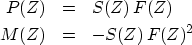(1) (2)
In Figure 1 it appears that F(Z) is nearly an impulse, whereas Figure 3 is dominated by the nonimpulsive aspect of F(Z). Algebraically the solutions of equations (1) and (2) are(3) (4)

These solutions can be computed in the Fourier domain. The difficulty is that the divisors in equations (3) and (4) can be zero, or small. This difficulty can be attacked by using a positive numberto stabilize it. Equation (3), for example, could be written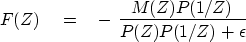(5)
We can easily understand what this means astends to infinity, where, because of the 1/Z, the matched filter has a noncausal response. Thus, although thestabilization seems nice, it apparently produces a nonphysical model. Forlarge or small, the time-domain response could turn out to be of much greater duration than is physically reasonable. This should not happen with perfect data, but in real life, data always has a limited spectral band of good quality.

Functions that are rough in the frequency domain will be long in the time domain. This suggests making a short function in the time domain by local smoothing in the frequency domain. Let the notationdenote smoothing by local averaging. Thus we can specify filters whose time duration is not unreasonably long by revising equation (5) to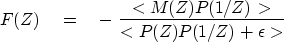(6)
where it remains to specify the method and amount of smoothing.

These time-duration difficulties do not arise in a time-domain formulation. First express (3) and (4) as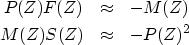(7) (8)
To imagine these in the time domain, refer back to equation (). Think ofwhereis a column vector containing the unknown sea-floor filter,is a column vector containing the portion of a seismogram in Figure 1 labeled multiple,'' andis a matrix of down-shifted columns, each column being the same as the signal labeled primary'' in Figure 1. The time-domain solutions are called shaping filters.'' For a simple filter of two coefficients, f0 and f1, we solved a similar problem, equation (), theoretically. With longer filters we use numerical methods.

In the time domain it is easy and natural to limit the duration and location of the nonzero coefficients in F(Z) and S(Z). The required program for this task is shaper(), which operates like cgmeth()and invstack()except that the operator needed here is contran().

# shaping filter
# minimize  SUM rr(i)**2  by finding ff and rr where
#
# rr = yy - xx (convolve) ff
#
subroutine shaper( nf,ff, nx,xx, ny, yy, rr, niter)
integer i, iter,   nf,    nx,    ny,         niter
real    ff(nf), xx(nx), yy(ny), rr(ny)
temporary real  df(nf), dr(ny), sf(nf), sr(ny)
if( ny != nx+nf-1)   call erexit('data length error')
do i= 1, nf
ff(i) = 0.
do i= 1, ny
rr(i) =  yy(i)
do iter= 0, niter {
call contran( 1, 0, nx,xx,  nf,df,  rr)         # df=xx*rr
call contran( 0, 0, nx,xx,  nf,df,  dr)         # dr=xx*df
call cgstep( iter, nf,ff,df,sf,  ny,rr,dr,sr)   # rr=rr-dr; ff=ff+df
}
return; end


The goal of finding the filters F(Z) and S(Z) is to best model the multiple reflections so that they can be subtracted from the data, enabling us to see what primary reflections have been hidden by the multiples. An important practical aspect is merging the analysis of many seismograms (see exercises).

Typical data includes not only that shown in Figures 1 and 3, but also wider source-receiver separation, as well as many other nearby shots and their receivers. Corrections need to be made for hyperbolic traveltime resulting from lateral separation between shot and receiver. Diffractions are a result of lateral imperfections in the generally flat sea floor. The spatial aspects of this topic are considered at great length in IEI. We will investigate them here in only a limited way.Next: Shaping a ghost to Up: SHAPING FILTER Previous: SHAPING FILTER
Stanford Exploration Project
10/21/1998